Documentation

## Design PID Controller Using Estimated Frequency Response

This example shows how to design a PI controller using a frequency response estimated from a Simulink model. This is an alternative PID design workflow when the linearized plant model is invalid for PID design (for example, when the plant model has zero gain).

### Open the Model

Open the engine control model and take a few moments to explore it.

```mdl = 'scdenginectrlpidblock'; open_system(mdl) ```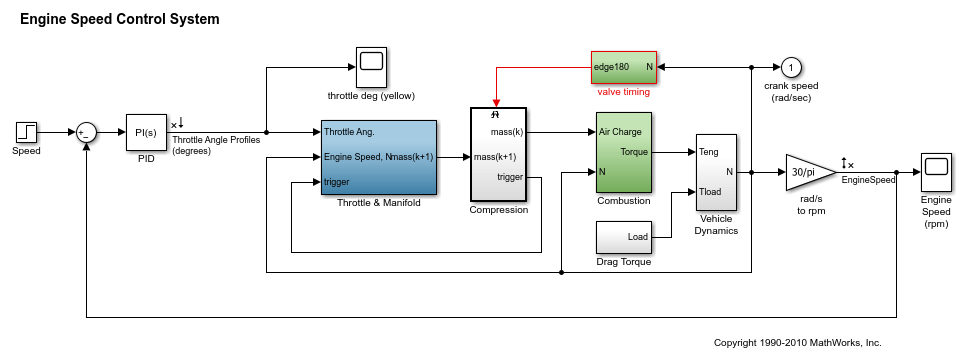The PID loop includes a PI controller in parallel form that manipulates the throttle angle to control the engine speed. The PI controller has default gains that makes the closed loop system oscillate. We want to design the controller using the PID Tuner that is launched from the PID block dialog.

```open_system([mdl '/Engine Speed (rpm)']) sim(mdl) ```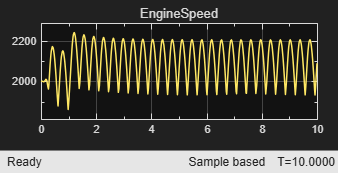Close the scope.

```close_system([mdl '/Engine Speed (rpm)']) ```

### PID Tuner Obtaining a Plant Model with Zero Gain From Linearization

In this example, the plant seen by the PID block is from throttle angle to engine speed. Linearization input and output points are already defined at the PID block output and the engine speed measurement respectively. Linearization at the initial operating point gives a plant model with zero gain.

To verify the zero linearization, first obtain the linearization input and output points from the model.

```io = getlinio(mdl); ```

Then, linearize the plant at its initial operating point.

```linsys = linearize(mdl,io) ```
```linsys = D = Throttle Ang EngineSpeed 0 Static gain. ```

The reason for obtaining zero gain is that there is a triggered subsystem (Compression) in the linearization path and the analytical block-by-block linearization does not support event-based subsystems. Since PID Tuner uses the same approach to obtain a linear plant model, *PID Tuner also obtains a plant model with zero gain and rejects it during the launching process.

To launch the PID Tuner, open the PID block dialog, and click Tune. An information dialog opens and indicates that the plant model linearized at the initial operating point has zero gain and cannot be used to design a PID controller.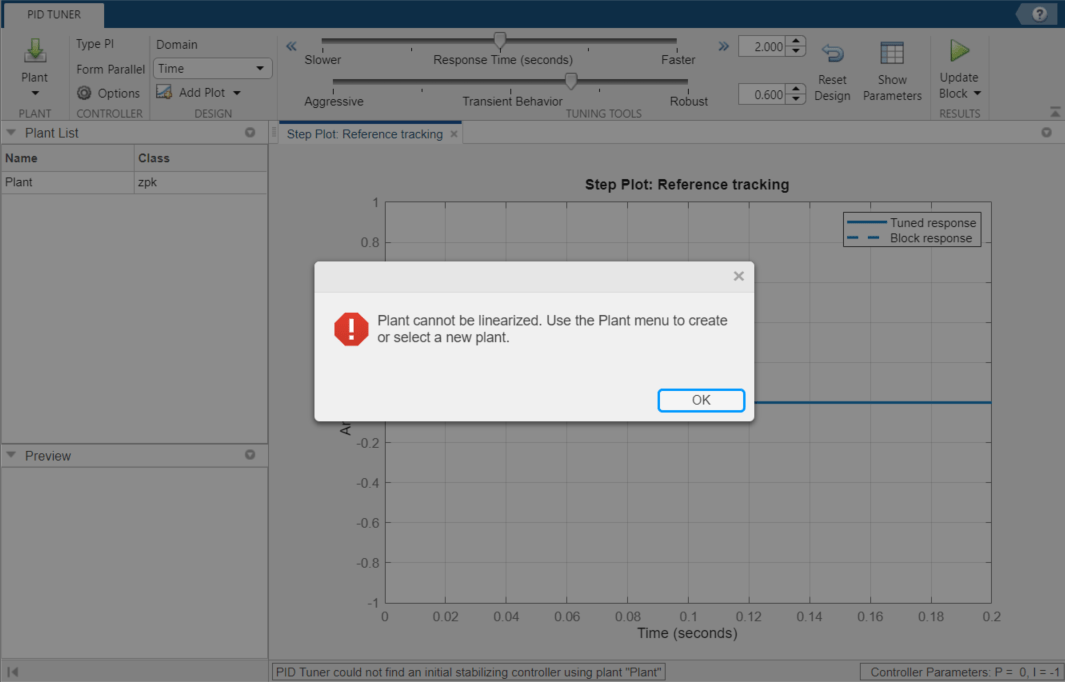An alternative way to obtain a linear plant model is to directly estimate the frequency response data from the Simulink model, create an `frd` system in the MATLAB workspace, and import it back to PID Tuner to continue PID design.

### Obtain Estimated Frequency Response Data Using Sinestream Signals

The sinestream input signal is the most reliable input signal for estimating an accurate frequency response of a Simulink model using the `frestimate` function. For more information on how to use `frestimate`, see Frequency Response Estimation Using Simulation-Based Techniques.

In this example, create a sine stream that sweeps frequency from 0.1 to 10 rad/sec with an amplitude of 1e-3. You can inspect the estimation results using the bode plot.

Construct the sinestream signal.

```in = frest.Sinestream('Frequency',logspace(-1,1,50),'Amplitude',1e-3); ```

Estimate the frequency response. This process can take a few minutes.

```sys = frestimate(mdl,io,in); ```

Display the estimated frequency response.

```bode(sys) ```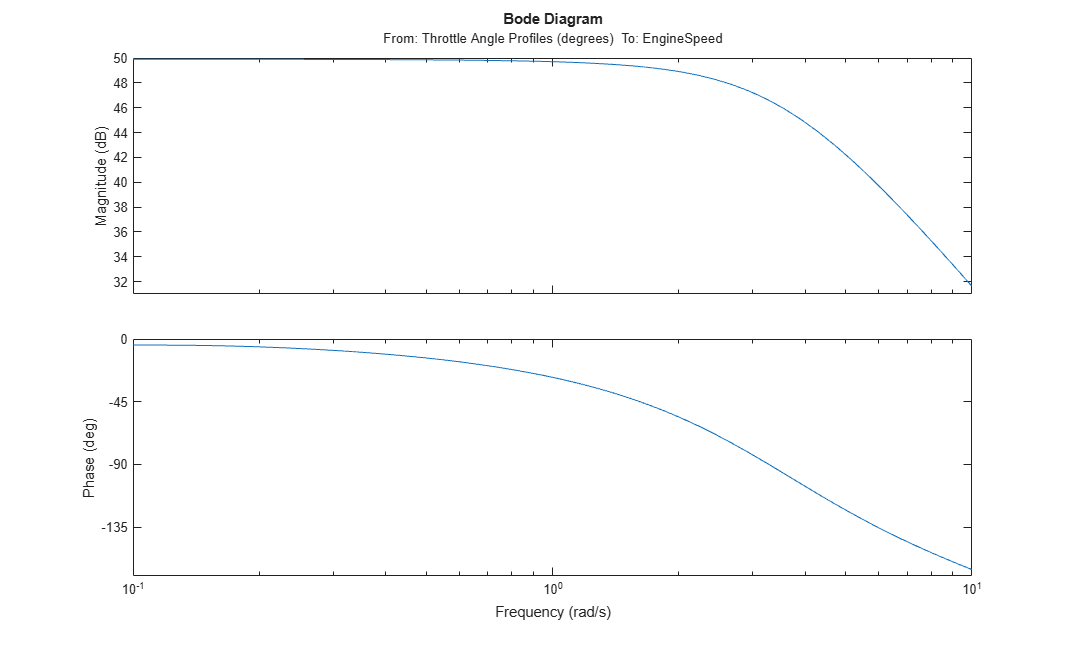### Design PI Controller

`sys` is an `frd` system that represents the plant frequency response at the initial operating point. To use it in PID Tuner, we need to import it after PID Tuner is launched. Click Plant, and select Import.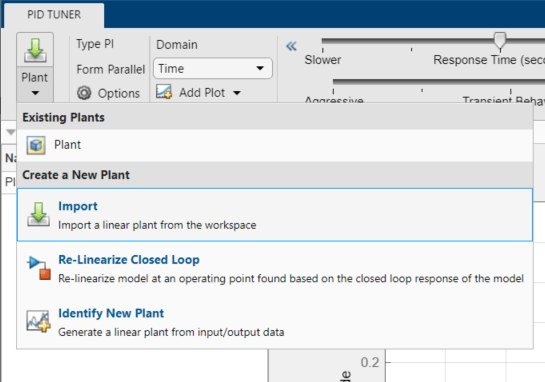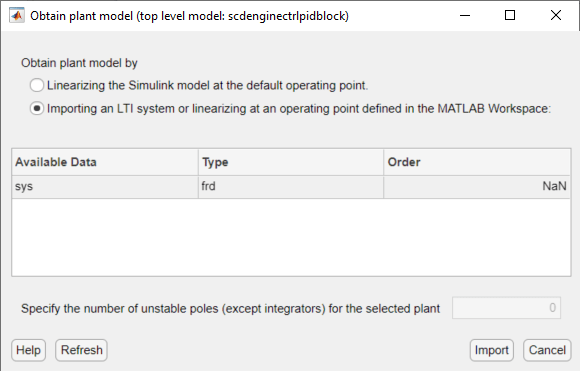Click Importing an LTI system, and in the list, select sys. Then, click "OK" to import the `frd` system into PID Tuner. The automated design returns a stabilizing controller. Click Add Plot, and select Open-Loop Bode plot. The plot shows reasonable gain and phase margin. Click Show Parameters to see the gain and phase margin values. Time domain response plots are not available for `frd` plant models.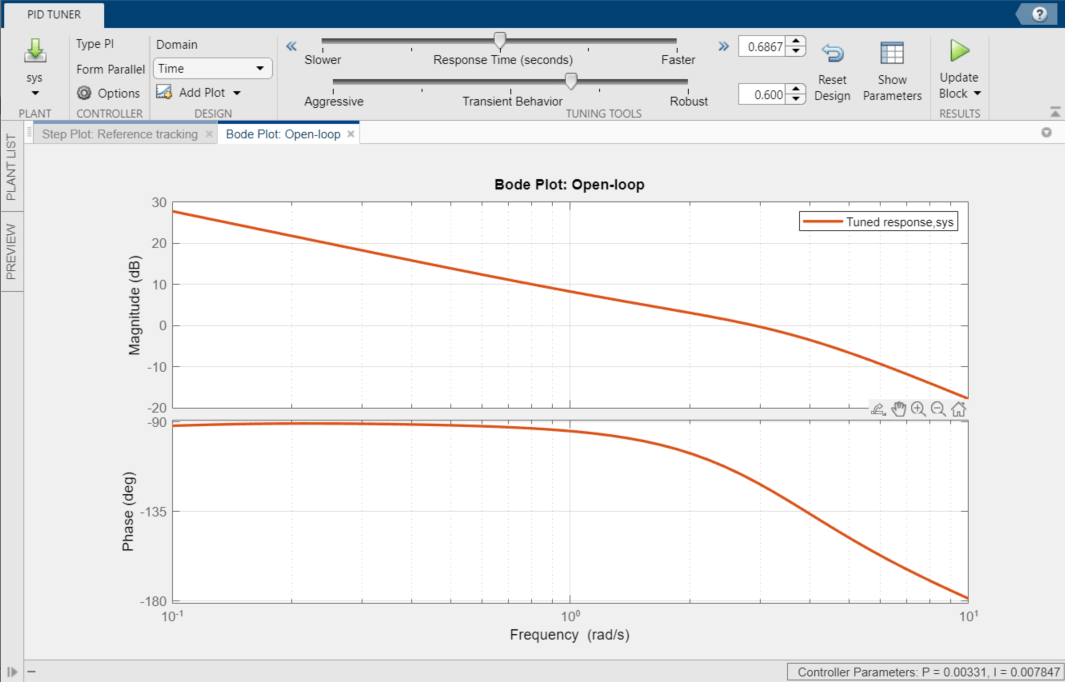To update the PID block P and I gains, click Update Block.

### Simulate Closed-Loop Performance in Simulink Model

Simulation in Simulink shows that the new PI controller provides good performance when controlling the nonlinear model.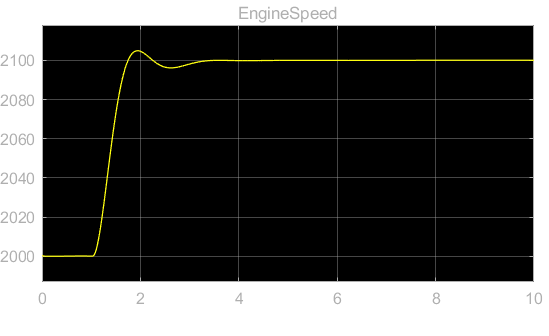Close the model.

```bdclose(mdl) ```